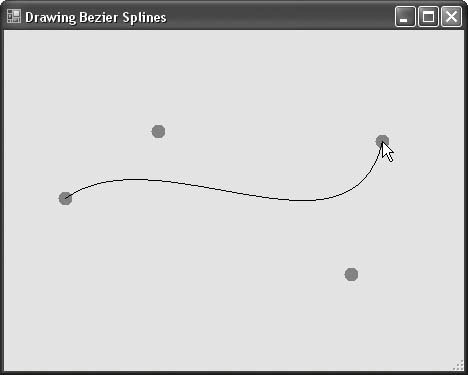# Recipe 9.14. Drawing Bezier Splines

#### Problem

You need to draw smooth curves between points, but you'd prefer not to delve into a lot of complex mathematical calculations.

#### Solution

Sample code folder: Chapter 09\BezierSplines

The DrawBezier() graphics method draws a smooth curve between two points, using two other points as control pointsor points that tug at the curve to change its shape as desired.

#### Discussion

Bezier splines are defined by two endpoints and two control points. (The mathematical theory behind Bezier splines is beyond the scope of this book. For more information, check out the links in the "See Also" section at the end of this recipe.)

The example program shown here lets you experiment interactively with the DrawBezier() graphics method. First, make sure you import the Drawing2D namespace, as follows:

` Imports System.Drawing.Drawing2D `

Up to four mouse-click points will be recorded in an array of points. Keep track of the points using a generic list:

` ' ----- Keeps track of the mouse positions. Dim BendPoints As New Generic.List(Of Point) `

As the mouse is clicked and new points are added to the array, the form is told to refresh itself by calling its Refresh() method:

` Private Sub Form1_MouseClick(ByVal sender As Object, _       ByVal e As System.Windows.Forms.MouseEventArgs) _       Handles Me.MouseClick    ' ----- Record another mouse position.    BendPoints.Add(New Point(e.X, e.Y))    ' ----- Update the display.    Me.Refresh() End Sub `

The form's Paint event is where the important action takes place:

` Private Sub Form1_Paint(ByVal sender As Object, _       ByVal e As System.Windows.Forms.PaintEventArgs) _       Handles Me.Paint    ' ----- Get the form's drawing surface.    Dim canvas As Graphics = e.Graphics `

Each point is drawn as a small solid-filled ellipse (circle). When there are four points, they are passed to the DrawBezier() method to draw the curve using a black pen. The first and fourth clicks are the endpoints. Clicking on the form a fifth time erases all the points, and the curve starts over:

`    Dim scanPoint As Point    Const PointSize As Integer = 7    ' ----- Draw available points.    If (BendPoints.Count <= 4) Then       For Each scanPoint In BendPoints          canvas.FillEllipse(Brushes.Red, _          scanPoint.X - PointSize, _          scanPoint.Y - PointSize, _          PointSize * 2, PointSize * 2)       Next scanPoint    End If    ' ----- Draw the spline if all points are there.    If (BendPoints.Count >= 4) Then       canvas.DrawBezier(Pens.Black, BendPoints(0), _          BendPoints(1), BendPoints(2), BendPoints(3))       BendPoints.Clear()    End If End Sub `

Figure 9-22 shows the results after four points have been clicked.

##### Figure 9-22. Drawing a Bezier splineVisual Basic 2005 Cookbook: Solutions for VB 2005 Programmers (Cookbooks (OReilly))
ISBN: 0596101775
EAN: 2147483647
Year: 2006
Pages: 400

Similar book on Amazon